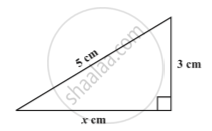Share

# Square Roots - Finding Square Roots

Course

#### notes

Study the following situations.
(a) Area of a square is 144 cm2. What could be the side of the square?
We know that the area of a square = "side"^2
If we assume the length of the side to be ‘a’, then 144 = a^2
To find the length of side it is necessary to find a number whose square is 144.
(b) In a right triangle the length of the hypotenuse and a side are respectively 5 cm and 3 cm in fig.Let x cm be the length of the third side.
Using Pythagoras theorem,
5^2 = x^2 + 3^2
25 – 9 = x^2
16 = x^2
Again, to find x we need a number whose square is 16.
Finding the number with the known square is known as finding the square root.

Finding square roots :
The inverse (opposite) operation of addition is subtraction and the inverse operation of multiplication is division. Similarly, finding the square root is the inverse operation of squaring.
We have,  1^2 = 1, therefore square root of 1 is 1
2^2 = 4, therefore square root of 4 is 2
3^2 = 9, therefore square root of 9 is 3 .

If you would like to contribute notes or other learning material, please submit them using the button below.